International
Tables for
Crystallography
Volume C
Mathematical, physical and chemical tables
Edited by E. Prince

International Tables for Crystallography (2006). Vol. C, ch. 6.3, pp. 599-600

## Section 6.3.1. Linear absorption coefficient

E. N. Maslena

aCrystallography Centre, The University of Western Australia, Nedlands, Western Australia 6009, Australia

### 6.3.1. Linear absorption coefficient

| top | pdf |

When a monochromatic X-ray beam of intensitytravels a distance T through a homogeneous isotropic material, the intensity is reduced to a value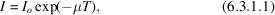where μ is the total linear absorption coefficient. This expression can also be applied to X-ray absorption in crystalline solids provided the absorption is insensitive to the arrangement of the atoms in the unit cell. This holds to a good approximation in most cases. If it is assumed that the absorption processes are additive,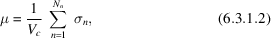whereis the cell volume and there are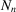contributions to absorption per cell.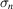is the absorption cross section for the nth contribution.

Of the processes that reduce the intensity of X-rays passing through matter, described in detail by Anderson (1984), photoelectric absorption, scattering, and extinction are important for the X-ray wavelengths used in crystallography.

#### 6.3.1.1. True or photoelectric absorption

| top | pdf |

In photoelectric absorption, X-ray photons disappear completely. The absorption of each photon results in the ejection from the atom of an electron that carries excess energy away as kinetic energy. The corresponding linear photoelectric absorption cross section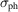is occasionally termed the cross section for `fluorescence'.

Excitation of an electron from a low to a higher bound state also occurs. Such an electron can be excited only if the photon energy exceeds the gap to the nearest unoccupied level. For this reason,varies abruptly in the manner shown in Fig. 6.3.1.1.Figure 6.3.1.1 | top | pdf |Idealized diagram showing the variation of the photoelectric absorption coefficient σph with wavelength λ.

The probability of ejection of an electron is largest for a photon energy just sufficient for excitation. It is small if the energy greatly exceeds that required. With increasing atomic number Z, the absorption edges shift to shorter wavelengths. The ratio of the value of σph for λ just below and just above the edge decreases with increasing Z, especially for the K edge.

The natural width of the resulting core-vacancy state sets a lower limit to the sharpness of the absorption edge (James, 1962). In some cases, such as the K edges for certain metals, the edge is substantially less sharp than that limit (Beeman & Friedman, 1939). Natural level widths are tabulated by Krause & Oliver (1979).

The wavelength of the absorption edge for a given element shifts slightly with changes in the chemical environment of the absorbing atom. There is also fine structure in the absorption coefficient that depends, especially on the short-wavelength side, both on chemical composition and on temperature. The range of the larger effects in the fine structure is of the order of 10−3 Å for X-ray wavelengths of approximately 1 Å. This corresponds to a photon-energy range of tens of electron volts, whereas X-ray photon energies are of the order of 10 keV. Smaller effects in the fine structure cover a far greater range. These are observed in extended X-ray absorption fine structure (EXAFS) spectra up to 1 keV from the edge (see Section 4.2.3). However, these small terms are of limited relevance when measuring X-ray diffraction intensities.

#### 6.3.1.2. Scattering

| top | pdf |

The X-ray photon is deflected from the original beam by collisions with atoms or electrons, the linear scattering cross section being σsc. The total cross section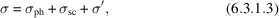where σ′ is the combined cross section for all processes other than photoelectric absorption or scattering.

There are two types of scattering process, coherent (Rayleigh) scattering and incoherent (Compton) scattering as described in Section 6.1.1. Rayleigh scattering may be regarded as resulting from a collision between a photon and an atom as a whole. Because the effective mass of a photon is far less than that of an atom, the photon retains its original energy. In a frame with the atom at rest, the scattering is elastic – i.e. the photon wavelength is essentially unmodified.

Rayleigh scattering by isolated atoms increases monotonically with Z – the cross section is proportional to the square of the integral of the atomic scattering factor f. However, the photoelectric absorption cross section increases far more rapidly, so Rayleigh scattering is relatively more important for atoms with low atomic number.

The atomic Rayleigh cross sections decrease with λ. Although there are anomalies near absorption edges, these have a limited effect on σ because the Rayleigh scattering in these regions is small compared with the photoelectric cross section except for the lightest elements.

The cross section for Compton scattering depends on the state of the electrons involved in the collision, but for very short wavelengths the atomic Compton cross section is approximately proportional to the atomic number. It varies far more slowly with λ than either the photoelectric or the Rayleigh cross section.

#### 6.3.1.3. Extinction

| top | pdf |

Because Rayleigh scattering is elastic, the scattering from different atoms may combine coherently, giving rise to interference, and hence to Bragg reflection from crystals.

For a crystal oriented so that there is no Bragg reflection, the interference reduces the scattered intensity far below the sum of the intensities that would be scattered by the atoms individually. For a strong Bragg reflection, on the other hand, the atomic scattering amplitudes add approximately in phase. The reduction in incident-beam intensity is many times larger than the sum of the squares of the individual atomic scattering powers.

An extreme case occurs in a perfect crystal, for which total reflection is possible. There is destructive interference with the incident beam producing a marked change in the index of refraction from its normal value of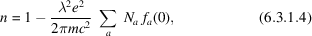where e and m are the charge and mass of the electron.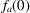is the scattering factor in the forward direction for an atom of type a and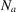is the number of atoms of that type per unit volume.

Thus, for strong reflections in near-perfect crystals, the Rayleigh scattering is affected by both crystal texture and beam direction. This reduction of primary-beam intensity due to the Rayleigh scattering is usually included, along with other specimen-dependent factors affecting diffracted-beam intensity, in the analysis of extinction.

#### 6.3.1.4. Attenuation (mass absorption) coefficients

| top | pdf |

Since the reduction of intensity depends on the quantity of matter traversed by the beam, the absorption coefficient is often expressed on a mass basis by dividing by the density ρm. μ/ρm defines the attenuation coefficient.

The determination of attenuation coefficients to high precision is possible only when contributions from all different scattering processes are analysed in detail. To a level of accuracy appropriate to most experiments, however, the coefficient can be determined from the atomic cross sections for scattering and photoelectric absorption. Ideally, absorption corrections for scattering from single crystals in the absence of extinction should be evaluated using the Rayleigh cross section for a crystal in the non-reflecting position. However, as Rayleigh scattering is a minor contribution to the total absorption except for the lighter elements, no large error is made by applying the absorption correction appropriate to an assembly of isolated atoms to a single crystal.

Likewise, μ/ρm is, to a good approximation, given by the sum of the attenuation coefficients for each constituent element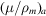, weighted by the mass fraction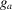for that element, i.e.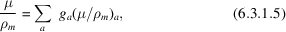where the sum is over the elements. The atomic cross section for attenuation is given by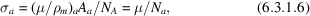where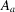is the atomic weight andis Avogadro's number. The evaluation of the attenuation coefficients is described in Section 4.2.4.

### References

Anderson, D. W. (1984). Absorption of ionizing radiation. Baltimore: University Park Press.
Beeman, W. W. & Friedman, H. (1939). The X-ray K absorption edges of the elements Fe (26) to Ge (32). Phys. Rev. 56, 392–405.
James, R. W. (1962). The optical principles of the diffraction of X-rays, pp. 135–192. Ithaca: Cornell University Press.
Krause, M. O. & Oliver, J. H. (1979). Natural widths of atomic K and L levels, Kα X-ray lines and several KLL Auger lines. J. Phys. Chem. Ref. Data, 8, 329–338.